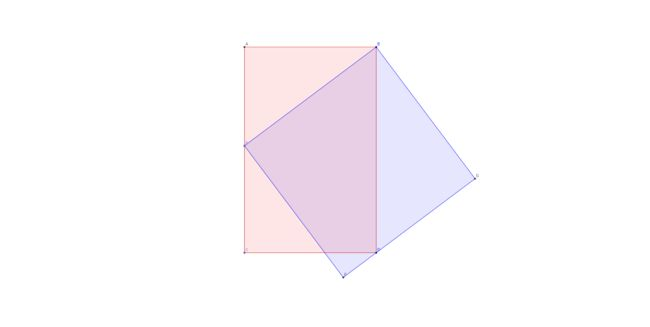# Nocturne No.1

Geometry Level 3The diagram shows a red rectangle with one of its vertices on one side of the square, and a blue square with one of its vertices on one side of the rectangle, both of which share a vertex.

Given that the length of the rectangle is 25 and the length of the square is 20, find the width of the rectangle.

×